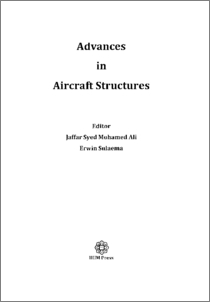# Accurate geometric stiffness matrix formulation of beam finite element

Sulaeman, Erwin (2011) Accurate geometric stiffness matrix formulation of beam finite element. In: Advances in Aircraft Structures. IIUM Press, Kuala Lumpur, pp. 190-197. ISBN ISBN 978-967-418-148-2Preview
PDF (Accurate geometric stiffness matrix formulation of beam finite element ) - Published Version

## Abstract

Alternate estimation of beam buckling load is presented in this Chapter. The standard procedure using a variational principle approach is usually utilized a cubic polynomial approach for the beam displacement shape such that the geometric stiffness matrix is a function of the geometric length of the element only. In the present work, the geometric stiffness matrix is developed as function geometric length and pre-assumed buckling load such that the accuracy of the buckling load can be improved by performing iteration. If the pre-assumed buckling load is set to zero, the present work will yield to the standard procedure. Therefore the present work offers a procedure to increase the accuracy of buckling estimation by performing iteration using the buckling load estimated by previous iteration as a good estimate for the pre-assumed buckling load.

Item Type: Book Chapter Buckling, geometric stiffness matrix, non-prismatic beam, finite element method T Technology > TL Motor vehicles. Aeronautics. Astronautics > TL500 Aeronautics Kulliyyah of Engineering > Department of Mechanical Engineering Dr Erwin Sulaeman 16 Jul 2012 21:47 16 Jul 2012 21:47 http://irep.iium.edu.my/id/eprint/22908View Item